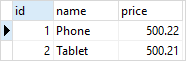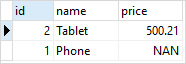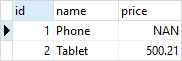# PostgreSQL Tutorial: NUMERIC Data Type

August 2, 2023

Summary: in this tutorial, you will learn about the PostgreSQL `NUMERIC` type for storing numeric data.

## Introduction to PostgreSQL NUMERIC data type

The `NUMERIC` type can store numbers with a lot of digits. Typically, you use the `NUMERIC` type for numbers that require exactness such as monetary amounts or quantities.

The following illustrate the syntax of the `NUMERIC` type:

``````NUMERIC(precision, scale)
``````

In this syntax, the `precision` is the total number of digits and the `scale` is the number of digits in the fraction part. For example, the number `1234.567` has the precision `7` and scale `3`.

The `NUMERIC` type can hold a value up to `131,072` digits before the decimal point `16,383` digits after the decimal point.

The scale of the `NUMERIC` type can be zero or positive. The following shows the syntax of `NUMERIC` type with scale zero:

``````NUMERIC(precision)
``````

If you omit both `precision` and `scale`, you can store any precision and scale up to the limit of the precision and scale mentioned above.

``````NUMERIC
``````

In PostgreSQL, the `NUMERIC` and `DECIMAL` types are equivalent and both of them are also a part of SQL standard.

If precision is not required, you should not use the `NUMERIC` type because calculations on `NUMERIC` values are typically slower than integers, floats, and double precisions.

## PostgreSQL NUMERIC examples

Let’s take some example of using the PostgreSQL `NUMERIC` type.

### 1) Storing numeric values

If you store a value with a scale greater than the declared scale of the `NUMERIC` column, PostgreSQL will round the value to a specified number of fractional digits.

See the following example.

First, create a new table named `products` for the demonstration:

``````DROP TABLE IF EXISTS products;

CREATE TABLE products (
id SERIAL PRIMARY KEY,
name VARCHAR(100) NOT NULL,
price NUMERIC(5,2)
);
``````

Second, insert some products with prices whose scales exceed the scale declared in the `price` column:

``````INSERT INTO products (name, price)
VALUES ('Phone',500.215),
('Tablet',500.214);
``````

Because the scale of the `price` column is 2, PostgreSQL rounds the value `500.215` up to `500.22` and rounds the value `500.214` down to `500.21` :

The following query returns all rows of the `products` table:

``````SELECT * FROM products;
``````In case you store a value whose precision exceeds the declared precision, PostgreSQL will raise an error as shown in the following example:

``````INSERT INTO products (name, price)
VALUES('Phone',123456.21);
``````

PostgreSQL issued the following error:

``````ERROR:  numeric field overflow
DETAIL:  A field with precision 5, scale 2 must round to an absolute value less than 10^3.
``````

### PostgreSQL NUMERIC type and NaN

In addition to holding numeric values, the `NUMERIC` type can also hold a special value called `NaN` which stands for not-a-number.

The following example updates the price of the product id 1 to `NaN` :

``````UPDATE products
SET price = 'NaN'
WHERE id = 1;
``````

Notice that you must use single quotes to wrap the `NaN` as shown in the `UPDATE` statement above.

The following query returns the data of the `products` table:

``````SELECT * FROM products;
``````Typically, the `NaN` is not equal to any number including itself. It means that the expression `NaN = NaN` returns false.

However, two `NaN` values are equal and `NaN` is greater than other numbers. This implementation allows PostgreSQL to sort `NUMERIC` values and use them in tree-based indexes.

The following query sorts the products based on prices:

``````SELECT * FROM products
ORDER BY price DESC;
``````As you can see from the output, the `NaN` is greater than `500.21`

In this tutorial, you have learned about the PostgreSQL `NUMERIC` data types and how to use `NUMERIC` column to store numbers.